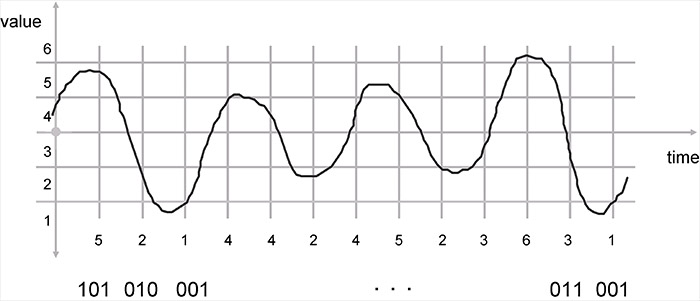# Telecommunications Tutorials

## Tutorial: Voice Digitization Principles

In this tutorial, we understand how voice is digitized - turned into a stream of 1s and 0s to be communicated over the IP packet-based telecommunications network.

This is a necessary step for carrying Voice over IP, which is taking over the world, and was used on older channelized SONET and T1 trunk carrier systems.There are three steps in voice digitization: quantization, sampling and coding:

• Quantization: Change from continuous in value to discrete in value
• Sampling: Change from continuous in time to discrete in time
• Coding: Code value of sample into 1s and 0s

Quantization is the process of changing from a signal which is continuous in value to a signal which is discrete in value.

This is accomplished by dividing the possible range of values into a number of "bins" or levels or steps, and assigning a number to each of these levels.

Then, when asked what the value of the signal is, we say that the signal is "in level #42" rather than measuring its voltage.

Another example of quantization is sugar cubes. Instead of putting some fractional value of a bag of sugar in your coffee, your choice is "one lump or two".

The sugar has been quantized into uniform lumps.

The second step is sampling. Sampling is the process of changing the signal from being continuous in time to one that is discrete in time.

On a regular basis, we take the value of the signal and record it. The value of the signal is the level number.

How often do we need to sample the signal?

A mathematician by the name of Nyquist proved that the signal has to be sampled more than twice as often as the frequency bandwidth of the signal to be able to reproduce it. This is called the Nyquist Rule.

The third step is coding. The value of the signal taken at each sample (the level number) must be coded into 1's and 0's so that it can be transmitted over a digital carrier system or stored in a computer.

At the far end, we perform the reverse process: re-creating the analog waveform from the received codes by de-coding the level number, generating a voltage with a value equal to that of the center of the level, and smoothly changing the voltage in this manner as each new code comes down the line.

The whole point in doing this is to move the analog voice signal from the near-end loop to the far-end loop without adding in any noise.

There is in fact a small amount of noise added in, up front, as part of the analog-to-digital conversion.

This is the quantization error, the difference in value between the center of the level, and where the signal actually was.

How do we make the quantization error smaller on average? Make the levels finer.

How many levels does the telephone company use? Enough so that a human can't hear the quantization error noise on the line.

Source: